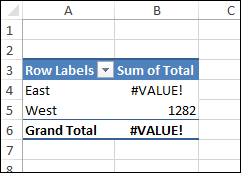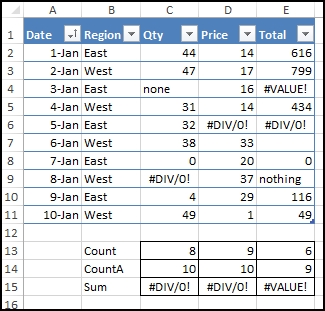# Errors in Pivot Table TotalsPivot tables are a quick and easy way to summarize a table full of data, without fancy formulas. Occasionally though, things can go wrong. Today we'll figure out why you might see errors in pivot table totals or subtotals, when all the item amounts look fine.

### Why Are There Errors?

Here's a simple pivot table with error values in the Grand Total row.It's understandable that the "Average of Price" total shows a #DIV/0! error, because that error also appears for the East region, in that column.

But why are there errors in the totals for the Count and Count Numbers columns? There aren't any errors in the amounts that are being totalled there.

### Check the Source Data

Some pivot table mysteries can be solved if you take a look at the source data, so let's start there.

Here's a screen shot of the small table that's the source for the pivot table. You can download this sample file from the Pivot Table Summary Functions page on my Contextures website.### Error Values in the Data

Obviously, there are some problems with that data.

• There is text in cell C4, so the formula in E4 has a #VALUE! error, instead of a numeric result
• There are three #DIV/0 errors, because Excel can't divide by zero
• There is a blank cell (E7), where a formula has been deleted
• Two cells (C4 and E9) contain text, instead of the numbers that should be in those columns

### Create a Pivot Table

What happens if you create a simple pivot table based on that error-filled data?

Things looked fine when I created this pivot table, with Region in the Rows area, and Count of Total in the Values area.

The error values have disappeared from the pivot table, and the counts for each Region are showing correctly.### Change to Sum Function

However, things take a turn for the worse if you change the Summary Function.

Instead of a Count of the Total amounts, try these steps to see a Sum.

• Right-click on one of the numbers in the Count of Total column
• Click Summarize Values by, and click Sum

As soon as the function changes to Sum, errors appear in the pivot table, for the East region, and in the Grand Total.### Just Like Worksheet Functions

The Pivot Table Sum function is like the worksheet SUM function, and it returns an error value, if there's one in the range being summed.

The Count function in a pivot table is like the worksheet COUNTA function. It counts text, numbers and errors, and does not count blank cells.

Pivot tables also have a Count Numbers function, which is like the worksheet COUNT function. It counts numbers, and does not count blank cells, errors or text.

### Totals in the Source Data

If you create Count, CountA and Sum totals in the source data, only the Sum totals show errors.Similarly, in the pivot table, Count and Count Numbers show totals.But Sum shows an error value.### Exceptions to the Error Rule

So, Count and Count Numbers shouldn't show error values in their Grand Totals.

And they don't, if they're all alone in the pivot table.

But, if you add another Value field, and it has errors, the Count and Count Numbers totals might suddenly show errors too.

You'll see errors in the subtotals and totals, if these 2 conditions are met:

• Oher summary functions are included in the pivot table, and those fields
contain errors
in the data
• There are error values in the data used for the Count and Count Number
columns

### No Errors in Data Field

Here's another look at the pivot table from the top of this post.The first 2 count columns have an error in the total, because:

• the Average of Price contains an error
• The Total data contains errors

However, the Count of Date column show a numeric total, because the source data doesn't have any errors in the Date field.

### Get the Sample File

You can download this sample file from the Pivot Table Summary Functions page on my Contextures website.

The zipped file is in xlsx format, and does not contain any macros.

____________________

### Errors in Pivot Table Totals______________________

This site uses Akismet to reduce spam. Learn how your comment data is processed.